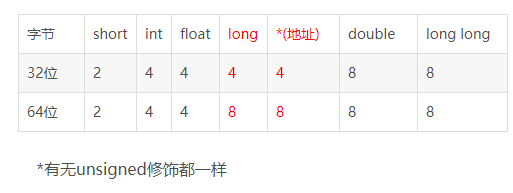# 深信服笔试

sizeof 的问题string s = "hello";
char str1[] = "Hello" ;
char str2 = {'H','e','l','l','o'};
char str3 = {'H','e','l','l','o','/0'};
char *p1 = "Hello";
char *p2[]={"hello","world"};
char *p3 = (char*)malloc(100);
char *p4 = (void*)malloc(100);
int n = 10;
int *q = &n;
char a = "abc\0def";

1. sizeof(s) = 32
对于String类型。每个编译器给分配内存的空间都是一定的，这里是32Byte。所以，不管字符串长度多长，内存分配都是32。当然也有4,16等情况，因编译期不同而不同。
2. sizeof (str1) = 6 (自动加了’/0’) strlen (str1 ) = 5 (字符串的长度)
3. sizeof (str2) = 5 (字符数组的大小) strlen (str2) = 未知 (该字符串缺少结束符’/0’)
4. sizeof (str3) = 6 (字符数组的大小) strlen (str3) = 5 (该字符串的长度为5)
5. sizeof (p1) = 4 (p1是一个指针，大小为4)
6. sizeof (p2) = 8 (p2是长度为2的字符串数组)
7. sizeof (p3) = 4 (p3是一个指针) sizeof(p4) = 4 (p4是一个指针)
8. sizeof (n) = 4 (整型大小为4)
9. sizeof (q) = 4 (q是一个指针，大小为4)
10. sizeof(a) = 10 (整个数组的长度) strlen(a) = 3 (字符串以‘\0’为结束标志，strlen只计算可见字符，而不会包含结束字符‘\0’)

void Function1( char p[],int num ){
cout << sizeof(p);
}
void Function2( int p[],int num ){
cout << sizeof(p);
}

1. 4 (数组在做为函数参数时均化为指针)
2. 4 (数组在做为函数参数时均化为指针)

class A{};
class B{
B(){};
~B(){};
};
class C:public A,public B{};
class D:virtual public A{};
class E:virtual public A,virtual public B{};

1. sizeof (A) = 1 (空类大小为1,编译器安插一个char给空类，用来标记它的每一个对象)
2. sizeof (B) = 1 (空类大小为1,编译器安插一个char给空类，用来标记它的每一个对象)
3. sizeof (C) = 1 (继承或多重继承后空类大小还是1)
4. sizeof (D) = 4 (虚继承时编译器为该类安插一个指向父类的指针，指针大小为4）
5. sizeof (E) = 8 (指向父类A的指针与父类B的指针，加起来大小为8）

class A{
public:
int a;
};
class B{
public:
int a ;
char b;
};
class C{
public:
int a ;
char b;
char c;
};


sizeof (A) = 4 （内含一个int ，所以大小为4）
sizeof (B) = 8 (int为4，char为1，和为5，考虑到对齐，总大小为int的整数倍即8)

class A{
public:
int a;
void Function();
};
class B{
public:
int a;
virtual void Function();
};
class C:public B{
public:
char b;
};
class D:public B{
public:
virtual void Function2();
};
class E{
public:
static void Function();
};


sizeof (A) = 4 (内含一个int，普通函数不占大小)
sizeof (B) = 8 (一个int，一个虚函数表指针)
sizeof (C) =12 (继承来一个int和一个虚函数表指针，一个char ,再加上数据对齐)
sizeof (D) = 8 (继承来一个int和一个虚函数表指针，多个虚函数是放在一个表里的，所以虚函数表指针只要一个就行了)
sizeof (E) = 1 (static 函数不占大小,空类大小为1)

class static_A
{
int static intVar;
static void fun(){}

};


sizeof (static_A) = 1 (静态数据成员不计入类内)

class A{
private:
int a;
};
class B:public A{};


sizof(B) = 4; (内含一个不可用的父类的int）

struct s1{};
struct s2
{
char c1;
int i;
char c2;
};
struct s3
{
char c1;
char c2;
int i;
};


1） 结构体变量的首地址能够被其最宽基本类型成员的大小所整除。

sizeof (s1) = 1 (空结构体也占内存)
sizeof (s2) = 12 sizeof(s3) = 8 (由此可见，结构体类型需要考虑到字节对齐的情况，不同的顺序会影响结构体的大小)


struct s1
{
short i;
struct
{
char c;
int j;
} ss;
int k;
};

struct stu5
{
char i;
struct
{
char c;
int j;
} ss;
char a;
char b;
char d;
char e;
char f;
};


1）展开后的结构体的第一个成员的偏移量应当是被展开的结构体中最大的成员的整数倍

2）结构体大小必须是所有成员大小的整数倍，这里所有成员计算的是展开后的成员，而不是将嵌套的结构体当做一个整体

sizeof (s1) = 16 (展开后最大的成员是ss.j大小为4)
sizeof (s2) = 20 ( 结构体ss单独计算占用空间为8，而stu5的sizeof则是20，不是8的整数倍，这说明在计算sizeof(stu5)时，将嵌套的结构体ss展开了，这样stu5中最大的成员为ss.j，占用4个字节，20为4的整数倍)


struct s
{
float f;
char p;
};


sizeof (s) = 20

double* (*a);
cout<<sizeof(a)<<endl; // 4
cout<<sizeof(*a)<<endl; // 72
cout<<sizeof(**a)<<endl; // 24
cout<<sizeof(***a)<<endl; // 4
cout<<sizeof(****a)<<endl; // 8

1. a是一个很奇怪的定义，他表示一个指向 double*类型数组的指针。既然是指针，所以sizeof(a) = 4
2. *a就表示一个元素为double*、大小为的多维数组类型，因此sizeof (*a) = 3*6*sizeof (double*) = 72
3. **a表示一个double*类型的数组，所以sizeof (**a) = 6sizeof (double) = 24
4. ***a就表示其中的一个元素，也就是double*了，所以sizeof (***a) = 4
5. ****a就是一个double了，所以sizeof (****a) = sizeof (double) = 8

N个X升容器，叠放在一起，如果某容器注水超过X升水，则溢出到下一层。给出每一层容器原来装有多少水，现注入多少水，求最终会有多少水溢出。注：注水时从底层开始往上走

N X

10 10
0 9962
1 2642
7 8773
9 523
6 3882
7 1093
0 3933
7 2276
6 4792
6 8904

46729

n, x =  map(int, input().split())
res, xx = 0, 0
for i in range(n):
v = x + xx
if ori + add > v:
res += ori + add - v
else:
xx = v - (ori + add)
print(res)


2 3

1

n, m = map(int, input().split())
l, res, k = [], [], 0
for i in range(1, n +1):
l.append(i)
print(l)
while len(res) != n:
for i in range(1, len(l) + 1):
num = k + i
if num % m == 0 or str(num).endswith(str(m)):
res.append(l[i -1])
l[i - 1] = 0
ll = [i for i in l if i !=0]
l = ll
k += i
print(res[-1])


5 2
316 861 5 38 483 235

861

#include <bits/stdc++.h>

using namespace std;

int flag = 0;
// 判断数组中是否k个数和为s的组合
void fun(vector<int> a, int cnt, int k, int i, int s)
{
int cnt_tmp = cnt;
if(s==0 && cnt==k) flag = 1;
if(i>=a.size()) return;
fun(a, cnt_tmp, k, i+1, s);
cnt_tmp ++;
fun(a, cnt_tmp, k, i+1, s-a[i]);
}

int main()
{
int n, m;
vector<int> price;
int cnt = 0;
int sum, sub_sum;
cin >> n >> m;
for(int i=0, tmp;i<=n;i++)
{

cin >> tmp;
price.push_back(tmp);

}
for(int i=0;i<=n;i++)
{
int tmp = price[i];
vector<int> pprice(price);
vector<int>::iterator it = pprice.begin() + i;
pprice.erase(it);
sum = accumulate(pprice.begin(), pprice.end(), 0);
if(sum%3==0)
{
sub_sum = sum/3*2;
flag = 0;
fun(price, cnt, m, 0, sub_sum);
if(flag)
{
cout << tmp;
return 0;
}
}
}
cout << "No";
return 0;
}


©️2019 CSDN 皮肤主题: 大白 设计师: CSDN官方博客